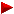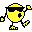HOME plateWON | World!OfNumbersJoe Crump found more Tridigital Squaresusing the 'Quadratic Residues' techniqueSquares containing at most three distinct digits

Introduction

A tridigital square is a square that contains at most three
distinct or unique squares like for instance with digits {2,6,9}
62969966996922666266229669292996
which is the square of 7935361806302386.

JoeCrump (email) was so kind to explain the quadratic
residues
technique that turned out to be very effective in
discovering more tridigital squares. I let him do the talking...

```Basically I iteratively test numbers of the form (10^A)x + y, where A
is a chosen number of digits. I build a list of candidate y's by testing
all the possible trailing A-digit combinations that satisfy the tridigital
property, and who are quadratic residues....

To explain quadratic residues consider the following. All numbers could be
represented as one of the following forms:

10x+0, 10x+1, 10x+2, 10x+3, 10x+4,
10x+5, 10x+6, 10x+7, 10x+8, 10x+9

If we square all these forms we find that no square can end with digits
2, 3, 7, or 8. If we do the same thing for 100x+c, c=0..99, we find
only the following can be the last two digits of squares...

00, 01, 04, 09, 16, 21, 24, 25, 29, 36, 41, 44,
49, 56, 61, 64, 69, 76, 81, 84, 89, 96

Quadratic residues allow you to quickly identify non squares. For this
problem, you could have also just iterated the numbers up to 10^A and
collected those whose square satisfied the tridigital property, but
quadratic residues come in handy in several places.

Anyway, I build of list of y, then for each y only test those x's where
the leading digits could satisfy the tridigital property. For instance,
let's say we're looking for squares with only digits 2,3,9 and we are
testing numbers of the form 1000x+177.
It only takes a glimpse at the calculations with increasing x to see
that we would be wasting a lot of tests on numbers that couldn't possibly
satisfy the equation.
I.e. x=100, we have 10035431329, x=101 we have 10236785329, etc. The target
not exceed an equal number of digits in sqrt(20/9) (1.490711985).

digits to shorten the range of numbers to test.

I have an idea though to possibly find HUGE solutions although I need to
research it to see if it could be fruitful. We can arbitrarily find large
numbers whose square starts with at least half of whatever digits we want.
For instance,
take the sqrt(20/9) = 1.490711985 and iteratively compute the square of
the first x digits rounded, i.e.

(14+1)^2 = 225
(149)^2 = 22201
(1491)^2 = 2223081
(14907)^2 = 222218649
(149071)^2 = 22222163041
etc.

We have squares that lead with all 2's. Now, all we need is to make the
trailing digits satisfy the property we're after or just iterate a ton of
these numbers until such a one falls into place.

Also, keep in mind that numbers close to these numbers also have the same
property so there is a good range to test. Furthermore, you'll find that
a lot of the solutions known are relatively close to the sqrt approximations
for the fraction representing repeating digits.

A good example is: 8819171038 squared = 77777777797497997444

The square root of 7/9 is 0.881917103688.... and you see how close it is
to the target number.

I'm excited about finding some possibly HUGE solutions (i.e. 100+ digits)
this way, but so far haven't done much with it. I did verify though of the
first 100 digit numbers the best solution I could find is the one above
from sqrt(7/9). It shows promise though as it required comparitively little
calculation.

Take care!

Joe Crump [ September 9, 2000 ]

```

References

```

```

[TOP OF PAGE]

Patrick De Geest - Belgium- Short Bio - Some Pictures